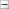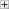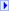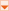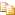Collapse AllExpand AllCode: All Code: Multiple Code: C# Code: Visual Basic Code: Visual C++Members: Show All Members: Filtered Members: Filtered Members: Filtered

[TCheckBoxFormControlObj]C#

[TCheckBoxFormControlObj]Visual Basic

[TCheckBoxFormControlObj]
Visual C++

[TCheckBoxFormControlObj]Include Protected Members

[TCheckBoxFormControlObj]
Include Inherited Members
 DPSF API Documentation DPSFDefaultQuadParticleSystem < (Of < ( < ' Particle, Vertex>)>)>..::..CInitialPropertiesForQuad Members<(Of <(<'Particle, Vertex>)>)>..::..CInitialPropertiesForQuad generic type exposes the following members.Constructors

 Name DescriptionInitializes a new instance of the DPSFDefaultQuadParticleSystem<(Of <(<'Particle, Vertex>)>)>..::..Methods

 Name DescriptionEquals Determines whether the specified Object is equal to the current Object. (Inherited from Object.)Finalize Allows an Object to attempt to free resources and perform other cleanup operations before the Object is reclaimed by garbage collection. (Inherited from Object.)GetHashCode Serves as a hash function for a particular type. (Inherited from Object.)GetType Gets the Type of the current instance. (Inherited from Object.)MemberwiseClone Creates a shallow copy of the current (Inherited from Object.)ToString Returns a String that represents the current Object. (Inherited from Object.)Fields

 Name DescriptionAccelerationMax (Inherited from DPSFDefaultBaseParticleSystem<(Of <(<'Particle, Vertex>)>)>..::..AccelerationMin (Inherited from DPSFDefaultBaseParticleSystem<(Of <(<'Particle, Vertex>)>)>..::..EndColorMax (Inherited from DPSFDefaultBaseParticleSystem<(Of <(<'Particle, Vertex>)>)>..::..EndColorMin (Inherited from DPSFDefaultBaseParticleSystem<(Of <(<'Particle, Vertex>)>)>..::..EndHeightMaxEndHeightMinEndSizeMax The Max End Size for the particle's EndWidth and EndHeight properties. NOTE: If this is greater than zero, this will be used instead of the EndWidthMax and EndHeightMax properties.EndSizeMin The Min End Size for the particle's EndWidth and EndHeight properties. NOTE: If this is greater than zero, this will be used instead of the EndWidthMin and EndHeightMin properties.EndWidthMaxEndWidthMinExternalForceMax (Inherited from DPSFDefaultBaseParticleSystem<(Of <(<'Particle, Vertex>)>)>..::..ExternalForceMin (Inherited from DPSFDefaultBaseParticleSystem<(Of <(<'Particle, Vertex>)>)>..::..FrictionMax (Inherited from DPSFDefaultBaseParticleSystem<(Of <(<'Particle, Vertex>)>)>..::..FrictionMin (Inherited from DPSFDefaultBaseParticleSystem<(Of <(<'Particle, Vertex>)>)>..::..InterpolateBetweenMinAndMaxAcceleration If true the Acceleration will be somewhere on the vector joining the Min Acceleration to the Max Acceleration. If false each of the XYZ components will be randomly calculated individually between the Min and Max Acceleration XYZ values. Default value is false. (Inherited from DPSFDefaultBaseParticleSystem<(Of <(<'Particle, Vertex>)>)>..::..InterpolateBetweenMinAndMaxColors If true a Lerp'd value between the Min and Max Colors will be randomly chosen. If false the RGBA component values will be randomly chosen individually between the Min and Max Color RGBA values. Default value is false. (Inherited from DPSFDefaultBaseParticleSystem<(Of <(<'Particle, Vertex>)>)>..::..InterpolateBetweenMinAndMaxExternalForce If true the External Force will be somewhere on the vector joining the Min External Force to the Max External Force. If false each of the XYZ components will be randomly calculated individually between the Min and Max External Force XYZ values. Default value is false. (Inherited from DPSFDefaultBaseParticleSystem<(Of <(<'Particle, Vertex>)>)>..::..InterpolateBetweenMinAndMaxPosition If true the Position will be somewhere on the vector joining the Min Position to the Max Position. If false each of the XYZ components will be randomly calculated individually between the Min and Max Position XYZ values. Default value is false. (Inherited from DPSFDefaultBaseParticleSystem<(Of <(<'Particle, Vertex>)>)>..::..InterpolateBetweenMinAndMaxRotation If true, the Rotation will be somewhere on the vector joining the Min Rotation to the Max Rotation. If false, each of the XYZ components will be randomly calculated individually between the Min and Max Rotation XYZ values. Default value is false.InterpolateBetweenMinAndMaxRotationalAcceleration If true, the Rotational Acceleration will be somewhere on the vector joining the Min Rotational Acceleration to the Max Rotational Acceleration. If false, each of the XYZ components will be randomly calculated individually between the Min and Max Rotational Acceleration XYZ values. Default value is false.InterpolateBetweenMinAndMaxRotationalVelocity If true, the Rotational Velocity will be somewhere on the vector joining the Min Rotational Velocity to the Max Rotational Velocity. If false, each of the XYZ components will be randomly calculated individually between the Min and Max Rotational Velocity XYZ values. Default value is false.InterpolateBetweenMinAndMaxVelocity If true the Velocity will be somewhere on the vector joining the Min Velocity to the Max Velocity. If false each of the XYZ components will be randomly calculated individually between the Min and Max Velocity XYZ values. Default value is false. (Inherited from DPSFDefaultBaseParticleSystem<(Of <(<'Particle, Vertex>)>)>..::..LifetimeMax (Inherited from DPSFDefaultBaseParticleSystem<(Of <(<'Particle, Vertex>)>)>..::..LifetimeMin (Inherited from DPSFDefaultBaseParticleSystem<(Of <(<'Particle, Vertex>)>)>..::..PositionIsAffectedByEmittersPosition If true the Emitter's Position will be added to the Particle's starting Position. For example, if the Particle is given an initial position of zero it will be placed wherever the Emitter currently is. Default value is true. (Inherited from DPSFDefaultBaseParticleSystem<(Of <(<'Particle, Vertex>)>)>..::..PositionMax (Inherited from DPSFDefaultBaseParticleSystem<(Of <(<'Particle, Vertex>)>)>..::..PositionMin (Inherited from DPSFDefaultBaseParticleSystem<(Of <(<'Particle, Vertex>)>)>..::..RotationalAccelerationMaxRotationalAccelerationMinRotationalVelocityMaxRotationalVelocityMinRotationMaxRotationMinStartColorMax (Inherited from DPSFDefaultBaseParticleSystem<(Of <(<'Particle, Vertex>)>)>..::..StartColorMin (Inherited from DPSFDefaultBaseParticleSystem<(Of <(<'Particle, Vertex>)>)>..::..StartHeightMaxStartHeightMinStartSizeMax The Max Start Size for the particle's StartWidth and StartHeight properties. NOTE: If this is greater than zero, this will be used instead of the StartWidthMax and StartHeightMax properties.StartSizeMin The Min Start Size for the particle's StartWidth and StartHeight properties. NOTE: If this is greater than zero, this will be used instead of the StartWidthMin and StartHeightMin properties.StartWidthMaxStartWidthMinVelocityIsAffectedByEmittersOrientation If true the Particle's Velocity direction will be adjusted according to the Emitter's Orientation. For example, if the Emitter is orientated to face backwards, the Particle's Velocity direction will be reversed. Default value is true. (Inherited from DPSFDefaultBaseParticleSystem<(Of <(<'Particle, Vertex>)>)>..::..VelocityMax (Inherited from DPSFDefaultBaseParticleSystem<(Of <(<'Particle, Vertex>)>)>..::..VelocityMin (Inherited from DPSFDefaultBaseParticleSystem<(Of <(<'Particle, Vertex>)>)>..::..See Also

DPSF NamespaceSend comments on this topic to